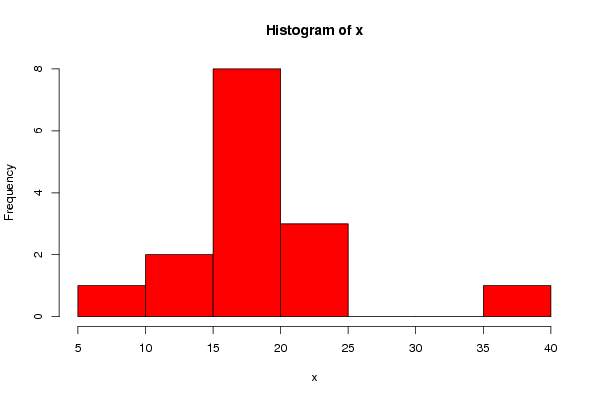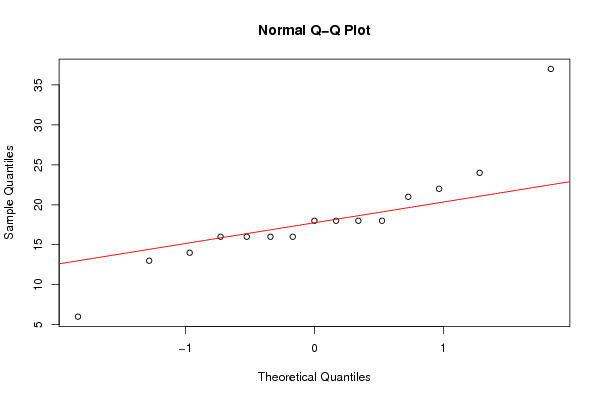Free Statistics

of Irreproducible Research!

Author's title
Author*The author of this computation has been verified*
R Software ModuleIan.Hollidayrwasp_varia1.wasp
Title produced by softwareHistogram and QQplot
Date of computationSun, 14 Mar 2010 14:13:23 +0100
Cite this page as followsStatistical Computations at FreeStatistics.org, Office for Research Development and Education, URL https://freestatistics.org/blog/index.php?v=date/2010/Mar/14/t1268572647b330o836t08u9l7.htm/, Retrieved Wed, 19 Jan 2022 10:24:17 +0000
Statistical Computations at FreeStatistics.org, Office for Research Development and Education, URL https://freestatistics.org/blog/index.php?pk=74386, Retrieved Wed, 19 Jan 2022 10:24:17 +0000
QR Codes:Original text written by user:
IsPrivate?No (this computation is public)
User-defined keywords
Estimated Impact126
Family? (F = Feedback message, R = changed R code, M = changed R Module, P = changed Parameters, D = changed Data)
-     [Histogram and QQplot] [PY2224 Aston Work...] [2009-10-06 09:57:09] [98fd0e87c3eb04e0cc2efde01dbafab6]
-    D    [Histogram and QQplot] [] [2010-03-14 13:13:23] [5b58052098f4b0433c56ae9d9a4453be] [Current]
-    D      [Histogram and QQplot] [] [2010-03-14 13:19:59] [e8bb49267f0b4e611f4778412d0811f2]
-    D      [Histogram and QQplot] [] [2010-04-19 12:21:33] [e8bb49267f0b4e611f4778412d0811f2]
Feedback Forum

Post a new message
Dataseries X:
6
24
21
18
16
37
16
18
18
13
22
16
18
16
14

 Summary of computational transaction Raw Input view raw input (R code) Raw Output view raw output of R engine Computing time 1 seconds R Server 'RServer@AstonUniversity' @ vre.aston.ac.uk

\begin{tabular}{lllllllll}
\hline
Summary of computational transaction \tabularnewline
Raw Input & view raw input (R code)  \tabularnewline
Raw Output & view raw output of R engine  \tabularnewline
Computing time & 1 seconds \tabularnewline
R Server & 'RServer@AstonUniversity' @ vre.aston.ac.uk \tabularnewline
\hline
\end{tabular}
%Source: https://freestatistics.org/blog/index.php?pk=74386&T=0

[TABLE]
[ROW][C]Summary of computational transaction[/C][/ROW]
[ROW][C]Raw Input[/C][C]view raw input (R code) [/C][/ROW]
[ROW][C]Raw Output[/C][C]view raw output of R engine [/C][/ROW]
[ROW][C]Computing time[/C][C]1 seconds[/C][/ROW]
[ROW][C]R Server[/C][C]'RServer@AstonUniversity' @ vre.aston.ac.uk[/C][/ROW]
[/TABLE]
Source: https://freestatistics.org/blog/index.php?pk=74386&T=0

Globally Unique Identifier (entire table): ba.freestatistics.org/blog/index.php?pk=74386&T=0

As an alternative you can also use a QR Code:

The GUIDs for individual cells are displayed in the table below:

 Summary of computational transaction Raw Input view raw input (R code) Raw Output view raw output of R engine Computing time 1 seconds R Server 'RServer@AstonUniversity' @ vre.aston.ac.uk

 Descriptive Statistics mean 18.2 formula standard deviation 6.66761897960155 formula

\begin{tabular}{lllllllll}
\hline
Descriptive Statistics \tabularnewline
mean & 18.2 & formula \tabularnewline
standard deviation & 6.66761897960155 & formula \tabularnewline
\hline
\end{tabular}
%Source: https://freestatistics.org/blog/index.php?pk=74386&T=1

[TABLE]
[ROW][C]Descriptive Statistics[/C][/ROW]
[ROW][C]mean[/C][C]18.2[/C][C]formula[/C][/ROW]
[ROW][C]standard deviation[/C][C]6.66761897960155[/C][C]formula[/C][/ROW]
[/TABLE]
Source: https://freestatistics.org/blog/index.php?pk=74386&T=1

Globally Unique Identifier (entire table): ba.freestatistics.org/blog/index.php?pk=74386&T=1

As an alternative you can also use a QR Code:

The GUIDs for individual cells are displayed in the table below:

 Descriptive Statistics mean 18.2 formula standard deviation 6.66761897960155 formulaPNG link Postscript link PDF linkPNG link Postscript link PDF link

Parameters (Session):
par1 = 10 ;
Parameters (R input):
par1 = 10 ;
R code (references can be found in the software module):
bitmap(file='test1.png')
par1 <- as.numeric(par1)
myhist<-hist(x, breaks=par1, col=2)
dev.off()
bitmap(file='test2.png')
qqnorm(x)
qqline(x, col=2)
dev.off()
a<-table.start()
a<-table.row.start(a)
a<-table.element(a,'Descriptive Statistics',3,TRUE)
a<-table.row.end(a)
a<-table.row.start(a)